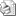## Abstracts, Extended Abstracts and the Conference Proceedings1. The "short abstracts" (1/2 page) uploaded via the registration window will help us to classify the talks according to the conference topics.
2. The 2-pages "extended abstracts", uploaded via the conference website, will be briefly reviewed and printed in the Conference Workbook, available by the conference opening. The "extended abstracts" for the Workbook may be prepared and submitted either in Russian or in English, following the style files and the sample files.
3. Besides the Conference Workbook, we plan to publish selected FPS'15 contributions in a special issue of the "Journal of Superconductivity and Novel Magnetism" (Springer). The manuscripts intended for journal publication, in English, should be submitted at the FPS'15 registration desk. The manuscripts for journal publication will be selected based on referee reviews. Detailed requirements for the manuscript preparation and the sample files will be posted later.

## Template of the extended abstracts

Manuscripts for the FPS'15 Workbook may be submitted either in Russian or in English. The Workbook (single volume, A4 page size) will be printed before the Conference opening and distributed among participants at the registration desk.

Manuscripts (as a tex-file supplemented with figure files) should be submitted before the deadline. The manuscript files should be uploaded via the personal page of a registered participant at the conference web-site.

Length of the manuscripts:

• Plenary talks - up to 4 pages.
• Invited talks - up to 3 pages.
• Oral talks and posters - up to 2 pages.

You may find the tex-file template below.

\documentclass[superscriptaddress,twocolumn,prl]{revtex4}

\usepackage{amssymb}
\usepackage{amsmath}
\usepackage{graphicx}
\usepackage{epsfig}
\usepackage[cp1251]{inputenc}

\usepackage[english]{babel}
%
% \usepackage[russian]{babel}
%

\begin{document}

\author{\underline{Presenting Author}}
\email{example@example.com}
\affiliation{Affiliation One}
\author{Author Two}
\affiliation{Affiliation Two}
\author{Author Three}
\affiliation{Affiliation One}
\affiliation{Affiliation Three}

\maketitle

The text of your abstract. It may contain several paragraphs, formulas.
Table~\ref{Petrov-table1} is an example of the reference.

\begin{table}[h]
\caption{\label{Petrov-table1}Table}
\begin{ruledtabular}
\begin{tabular}{ll}
Cell 1 & Cell 2
\end{tabular}
\end{ruledtabular}
\end{table}

Look at the example of the formula. Reference is~(\ref{Petrov-eq1}).

\begin{equation}
\cos^2\alpha + \sin^2\alpha \approx 1,
\label{Petrov-eq1}
\end{equation}

For several formulas use align:
\begin{align}
i \hbar \frac{\partial}{\partial t} \Psi &= \hat{H} \Psi \\
i\hbar\frac{\partial}{\partial t} \Psi(\vec{r} ,t) &=
\left [ -\frac{\hbar^2}{2m}\nabla^2 + V(\vec{r},t)\right ] \Psi(\vec{r} ,t)
\end{align}

To hyphenate formula one can use multline environment:
\begin{multline}
G_{sH} = G_n + \int\left[\alpha |\Psi|^2 + \frac{\beta}{2} |\Psi|^4 + \right. \\
+ \left. \frac{1}{4m} \left| - i \hbar \nabla \Psi -
\frac{2e}{c} \mathbf{A} \Psi \right|^2 + \right. \\
+ \left. \frac{(\operatorname{rot} \mathbf{A})^2}{8\pi} -
\frac{\operatorname{rot} \mathbf{A} \cdot \mathbf{H_0} }{4\pi} \right] \mathrm{d} V
\end{multline}

\begin{figure}[h]
\begin{center}
\includegraphics[width=0.5\linewidth]{example-image}
\caption{Image example}
\end{center}
\end{figure}

And a list of references produced by the standard REV\TeX\  environment.